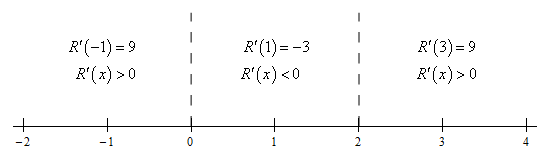Paul's Online Notes
Home / Calculus I / Derivatives / Differentiation Formulas
Show Mobile Notice Show All Notes Hide All Notes
Mobile Notice
You appear to be on a device with a "narrow" screen width (i.e. you are probably on a mobile phone). Due to the nature of the mathematics on this site it is best views in landscape mode. If your device is not in landscape mode many of the equations will run off the side of your device (should be able to scroll to see them) and some of the menu items will be cut off due to the narrow screen width.

### Section 3.3 : Differentiation Formulas

19. Determine where the function $$R\left( x \right) = \left( {x + 1} \right){\left( {x - 2} \right)^2}$$ is increasing and decreasing.

Show All Steps Hide All Steps

Hint : Recall the various interpretations of the derivative. One of them is exactly what we need to get the problem started.
Start Solution

Recall that one of the interpretations of the derivative is that it gives the rate of change of the function. Since we are talking about where the function is increasing and decreasing we are clearly talking about the rate of change of the function.

So, we’ll need the derivative. First however we’ll need to multiply out the function so we can actually take the derivative. Here is the rewritten function and the derivative.

$R\left( x \right) = {x^3} - 3{x^2} + 4\hspace{0.5in}\require{bbox} \bbox[2pt,border:1px solid black]{{R'\left( x \right) = 3{x^2} - 6x = 3x\left( {x - 2} \right)}}$

Note that the derivative was factored for later steps and doesn’t really need to be done in general.

Hint : Where is the function not changing?
Show Step 2

Next, we need to know where the function is not changing and so all we need to do is set the derivative equal to zero and solve.

$R'\left( x \right) = 0\hspace{0.5in} \Rightarrow \hspace{0.5in}3x\left( {x - 2} \right) = 0$

From this it is pretty easy to see that the derivative will be zero, and hence the function will not be moving, at,

$\require{bbox} \bbox[2pt,border:1px solid black]{{x = 0 \hspace{0.25in} x = 2}}$
Hint : How does the increasing/decreasing behavior of the function relate to the sign (positive or negative) of the derivative?
Show Step 3

To get the answer to this problem all we need to know is where the derivative is positive (and hence the function is increasing) or negative (and hence the function is decreasing). Because the derivative is continuous we know that the only place it can change sign is where the derivative is zero. So, as we did in this section a quick number line will give us the sign of the derivative for the various intervals.

Here is the number line for this problem.From this we get the following increasing/decreasing information.

\require{bbox} \bbox[2pt,border:1px solid black]{\begin{align*}{\mbox{Increasing :}} & \,\, - \infty < x < 0,\,\,\,\,\,2 < x < \infty \\ {\mbox{Decreasing :}} & \,\,0 < x < 2\end{align*}}